Ch 7. Centroid/Distributed Loads/Inertia Multimedia Engineering Statics Centroid: Line Area Vol Centroid: Composite Distributed Loads Area Moment of Inertia
 Chapter 1. Basics 2. Vectors 3. Forces 4. Moments 5. Rigid Bodies 6. Structures 7. Centroids/Inertia 8. Internal Loads 9. Friction 10. Work & Energy Appendix Basic Math Units Sections Search eBooks Dynamics Fluids Math Mechanics Statics Thermodynamics Author(s): Kurt Gramoll ©Kurt GramollSTATICS - THEORY Integrals of the form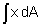are called first moments of the area. Accordingly, integrals of the form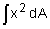are called second moments of the area, or area moments of inertia. Moments of Inertia for a Plane Area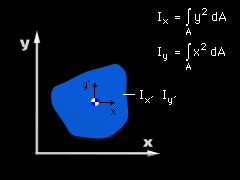Moment of Inertia for a Plane Area For an area A that lies in the x-y plane as shown, the area moments of inertia about the x and y axes are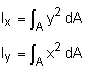Moments of inertia that are calculated about the centroid of the area are denoted      Ix'     Iy' The moments of inertia for basic shapes are tabulated in Sections Appendix. Polar Moment of Inertia for a Plane Area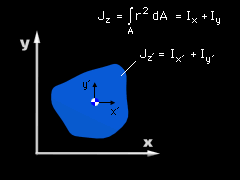Polar Moment of Inertia for a Plane Area The moment of inertia for an area that lies in the x-y plane can also be calculated about the z axis, which is known as the polar moment of inertia. The polar moment of inertia of the area A is calculated as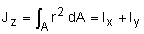If the polar moment of inertia is calculated at the centroid of the area, it is denoted      Jz' = Ix' + Iy' The polar moment of inertia is commonly used when calculating the torsion of shafts. Parallel Axis Theorem for an Area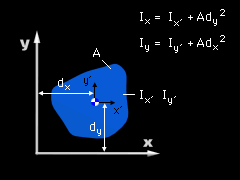Parallel Axis Theorem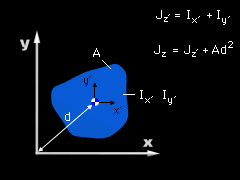Parallel Axis Theorem (Polar Moment of Inertia) If the area moments of inertia about the centroid are known, then the moments of inertia about any other parallel axis can be found as      Ix = Ix' + Ady2      Iy = Iy' + Adx2      Iz = Iz' + Ad2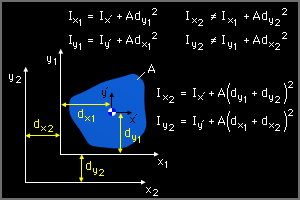Parallel Axis Theorem from the Centroid Moments of Inertia for Composite Areas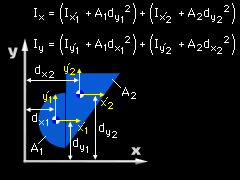Moment of Inertia - Composite Parts Just as the centroid of an area can be calculated by breaking the area into simpler composite parts, the moments of inertia of a complicated area can be calculated by breaking the area into simpler composite parts. For an arbitrary axis, the moments of inertia for an area made of composite parts are given by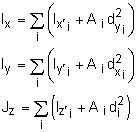This technique also works for polar moment of inertia. Subtraction of Material (Holes) Previously, in the Centroid: Composite Parts section, it was shown that when the centroid of line, area, or volume are calculated, holes or cutouts can be accounted for by considering them a negative line length, area, or volume respectively. When calculating moments of inertia, we can deal with cutouts and holes in the same manner. Both the moments of inertia about the centroid of the hole as well as the area of the hole are considered negative when we are summing the composite parts.

Practice Homework and Test problems now available in the 'Eng Statics' mobile app
Includes over 500 problems with complete detailed solutions.
Available now at the Google Play Store and Apple App Store.